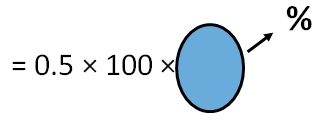Converting decimal to percent

Chapter 8 Class 7 Comparing Quantities
Concept wise

Suppose I have decimal

0.5

To continue into percentage

We just multiplying by 100%

0.5 = 0.5 × 100%

= 5/10 × 100%

= 5 × 10%

= 50%

Note:-

The concept behind this is

Percentage is 1/100

That is 1/100 = %

So, to convert any number into percentage,

We multiply by 100/100

Like here

0.5 = 0.5 × 100/100= 0.5 × 100 %

= 5/10 × 100%

= 5 × 10%

= 50%

Let’s do some examples

#### Convert 1.125 into percent

1.125 = 1.125 × 100%

= 1125/1000 × 100%

= 1125/10 %

= 112.5%

#### Convert 0.66 into percent

0.66 = 0.66 × 100%

= 66/100 × 100%

= 66/1 %

= 66%

Get live Maths 1-on-1 Classs - Class 6 to 12